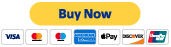*This was an attempted proof based on inverse fourier Transforms. Since then, a new proof was developed based on the Dirichlet Eta function. It can be found here.

Here is a series of ordered Equation Panels showing a method for and attempt at using Inverse Fourier Transforms along with the Dirichlet Eta function to explain the Riemann Hypothesis.

The first 3 panels show Wikipedia and Wolfram's statements of the hypothesis along with the Fourier Transform Equations. The 2 statements of the hypothesis vary slightly, but represent 2 of the most common ways it is presented.  This variation in statements regularly leads to misunderstandings in people that are new to the Riemann Hypothesis, specifically why both are presented, but once understood, reveals the logic for the Dirichlet Eta Equivalency Statement of the Riemann Hypothesis.

Starting then from the 4th panel and the Dirichlet Eta Equivalency Statement, the first step is to expand and divide the complex portion in the Dirichlet Eta so that the real and imaginary portion, a and b, of the complex input s can be separated.  This is completed and restated in panel 10.

One may now observe that the expanded and separated Dirichlet Eta is identical to a Fourier transform without an a-sub-0 term. This raises the question whether a wave can be found with a 1 to 1 correspondence, or bijection, to the Dirichlet Eta. Panels 11 to 23 show the process to obtain such a wave.  First taken, are the inverses of the a-sub-n and b-sub-n summations. This leads to 2 separate equations as the original f(x). However, since it must be the same f(x), both equations are set equal.

At this stage, there is now direct relation between a, the real portion, b, the imaginary portion, and x, the generator for all possible relations between a and b. This allows the nature of all possible a to be stated and examined in a governing functional form.

Next, panels 24 to 27 show that the only places a could have a non 0 value at its integers on its governing function are at one of 2 limits. The 1st limit equals 0, and the other equals 1/2, thus showing that the real portion can only be equal to 1/2. Panel 28 shows b in terms of x, instead of x in terms of b, which is part of the relationship between a, b, and x previously described.

Lastly, in regards to the overall technique and the utility of the created bijection, extra attention should be given to the nature of the governing function of a and the manner in which its values and limits are calculated. There was some ambiguity to me as to whether the bijection of the governing function is between its integer values and a, or between its real values and a. Either way, it shows that the governing function of a should be understood to have the following properties.  If the bijection is between the integers, then the function proves Riemann and is as was mentioned. If the bijection is between the reals, then the function disproves Riemann and then provides the would be other values of the real portion. Finally, further discussion of the a-sub-0 term after the inverse function is calculated, and how it has been or should be handled, may also be relevant.© 2017- 2020 by Thoughtfarm. Proudly created with Wix.com

This site was designed with the
.com
website builder. Create your website today.